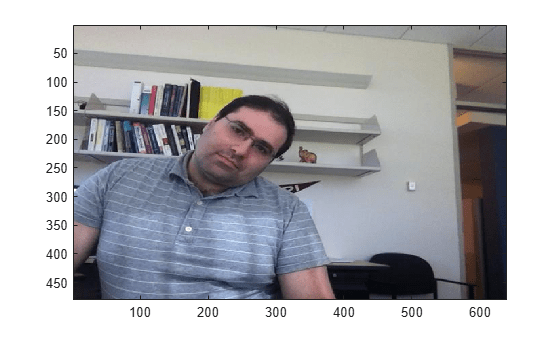Perform Face Detection by Using OpenCV in MATLAB

This example shows how to detect faces in an image or video by using prebuilt MATLAB® interface to the OpenCV function cv::CascadeClassifier. This example uses a Harr face detection model that is trained for scale-invariant, frontal face detection. In this example, you also use the createMat utility function to define the input and output arrays, the getImage utility function to read the output image returned by the OpenCV function, and the rectToBbox utility function to convert the face detection output returned by the OpenCV function to bounding box coordinates in MATLAB®.

Read a video into the MATLAB workspace by using the VideoReader MATLAB function.

Add the MATLAB interface to OpenCV package names to the import list.

import clib.opencv.*;
import vision.opencv.util.*;

Specify the file name of a pre-trained trained Haar face detection model.

Specify the scale factor to use for multi-scale detection.

scaleFactor = 1.2;

Follow these steps to detect faces in each frame by using the detectMultiScale method of the OpenCV class cv.CascadeClassifier.

• Create a Mat object and store the input frame to the Mat object by using the createMat function. Specify the Mat object as an input to the detectMultiScale method.

• Create a MATLAB array to represent the OpenCV class for 2D rectangles cv::Rect2i. Specify the array as an input to the detectMultiScale method. The method uses the array to return the detection results.

• Export the detection results returned by the detectMultiScale method to a row vector by using the rectToBbox function. The row vector specifies bounding box coordinates in one-based indexing.

• Draw the bounding boxes on the input frame to represent the detected faces.

count = 1;
detections = cell(1,videoSample.NumFrames);
while(hasFrame(videoSample))

[inputMat,inputArray] = createMat(testFrame);
results = clibArray("clib.opencv.cv.Rect2i", 0);

if results.Dimensions ~= 0
detections{count} = rectToBbox(results);
else
detections{count} = [];
end
testFrame = insertShape(testFrame,Rectangle=detections{count},LineWidth=5);
image(testFrame,Parent=gca);
pause(0.01)
count = count+1;
end